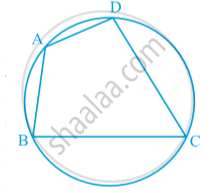#### notesA quadrilateral ABCD is called cyclic if all the four vertices of it lie on a circle in fig.  A quadrilateral is said to be a cyclic quadrilateral, if there is a circle passing through all its four vertices.
=>∠A + ∠C = 180°
also ∠B + ∠D = 180°.

#### theorem

Theorem: The sum of either pair of opposite angles of a cyclic quadrilateral is 180º.
Given : A cyclic quadrilateral ABCD .
To Prove : ∠BAD + ∠BCD = ∠ABC + ∠ADC = 180º.
Construction : Draw AC and DB.
Proof : ∠ACB = ∠ ADB  and
∠BAC = ∠BDC [Angles in the same segment]
therefore ∠ACB + ∠BAC = ∠ADB +∠BDC = ∠ADC
Adding ∠ABC on both the sides, we get
∠ACB + ∠BAC +∠ABC = ∠ADC +∠ABC
But ∠ACB +∠BAC +∠ABC = 180º.   [sum of the angles of a triangle]
therefore ∠ ADC + ∠ABC =180º.
therefore ∠BAD +∠BCD = 360º  - (∠ADC + ∠ABC) = 180º.
Hence proved.

Theorem: If the sum of a pair of opposite angles of a quadrilateral is 180º, the quadrilateral is cyclic.

If you would like to contribute notes or other learning material, please submit them using the button below.

#### Video Tutorials

We have provided more than 1 series of video tutorials for some topics to help you get a better understanding of the topic.

Series 1

Series 2